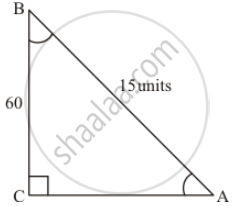Share
Notifications

View all notifications
Books Shortlist
Your shortlist is empty

# In Right Angled Triangle Abc. ∠C = 90°, ∠B = 60°. Ab = 15 Units. Find Remaining Angles and Sides. - CBSE Class 10 - Mathematics

Login
Create free account

Forgot password?
ConceptTrigonometric Ratios of an Acute Angle of a Right-angled Triangle

#### Question

In right angled triangle ABC. ∠C = 90°, ∠B = 60°. AB = 15 units. Find remaining angles and sides.

#### Solution 1

We are given the following triangle with related informationIt is required to find ∠A, ∠C and length of sides AC and BC

ΔABC is right angled at C

Therefore,

∠C = 90^@

Now we know that sum of all the angles of any triangle is 180^@

Therefore

∠A + ∠B + ∠C = 180^@

Now by substituting the values of known angles and in equation (1)

We get,

∠A + 60^@ + 90^2 = 180^@

Therefore

∠A + 150^@ = 180^@

=> ∠A = 180^@ - 150^@

=> ∠A = 30^@

Therefore,

∠A = 30^@

Now

We know that,

cos B = cos 60^@

=> (BC)/(AB) = cos 60^@

Now we have,

AB =15 units and cos 60^@ = 1/2

Therefore by substituting above values in equation (2)

We get,

cos B = cos 60^@

=> (BC)/(AB) = cos 60^@

=> (BC)/15 = 1/2

Now by cross multiplying we get,

(BC)/15 = 1/2

=> 2 xx BC = 15 xx 1

=> BC = 15/2

=> BC = 7.5

Therefore

BC = 7.5 units ....(3)

Now

We know that

sin B = sin 60^@

=> (AC)/(AB) = sin 60^@     .........(4)

Now

We know that,

AB=15 units and

#### Solution 2

We are given the following triangle with related informationIt is required to find ∠A, ∠C and length of sides AC and BC

ΔABC is right angled at C

Therefore,

∠C = 90^@

Now we know that sum of all the angles of any triangle is 180^@

Therefore

∠A + ∠B + ∠C = 180^@

Now by substituting the values of known angles and in equation (1)

We get,

∠A + 60^@ + 90^2 = 180^@

Therefore

∠A + 150^@ = 180^@

=> ∠A = 180^@ - 150^@

=> ∠A = 30^@

Therefore,

∠A = 30^@

Now

We know that,

cos B = cos 60^@

=> (BC)/(AB) = cos 60^@

Now we have,

AB =15 units and cos 60^@ = 1/2

Therefore by substituting above values in equation (2)

We get,

cos B = cos 60^@

=> (BC)/(AB) = cos 60^@

=> (BC)/15 = 1/2

Now by cross multiplying we get,

(BC)/15 = 1/2

=> 2 xx BC = 15 xx 1

=> BC = 15/2

=> BC = 7.5

Therefore

BC = 7.5 units ....(3)

Now

We know that

sin B = sin 60^@

=> (AC)/(AB) = sin 60^@     .........(4)

Now

We know that,

AB=15 units and sin 60^@ = sqrt3/2

Therefore by substituting above values in equation (4)

We get,

sin B = sin 60^@

=> (AC)/(AB) = sin 60^@

=> (AC)/15 = sqrt3/2

Now by cross multiplying we get,

=> 2 xx AC = sqrt3 xx 15

=> AC = (sqrt3 x 15)/2

=> AC = 15/2 sqrt3

Therefore,

AC = 15/2 sqrt3 units

Hence

A = 30^@

BC = 7.5untis

AC = 15/2 sqrt3 units

Is there an error in this question or solution?

#### APPEARS IN

Solution In Right Angled Triangle Abc. ∠C = 90°, ∠B = 60°. Ab = 15 Units. Find Remaining Angles and Sides. Concept: Trigonometric Ratios of an Acute Angle of a Right-angled Triangle.
S